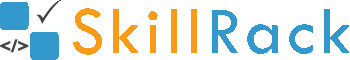Largest among N Values2 months ago

The program must accept N integers as the input. The program must print the largest integer among the N integers as the output.

Boundary Condition(s):
2 <= N <= 100
-1000 <= Each integer value <= 1000

Input Format:
The first line contains N.
The second line contains N integers separated by a space.

Output Format:
The first line contains the largest integer among the N integers.

Example Input/Output 1:
Input:
5
12 98 65 121 78

Output:
121

Explanation:
Here N = 5.
The largest integer among the 5 integers is 121.
Hence the output is 121

Example Input/Output 2:
Input:
4
-123 -9 -456 -3

Output:
-3

The below video explains the logic and the implementation in C programming language to find the largest among N values.

#include <stdio.h>
#include <stdlib.h>

int main()
{
int N;
scanf("%d", &N);
int largest, curr;
scanf("%d", &largest);
for(int ctr = 2; ctr <= N; ctr++)
{
scanf("%d", &curr);
if(curr > largest)
{
largest = curr;
}
}
printf("%d", largest);
return 0;
}

#include <iostream>

using namespace std;

int main()
{
int N;
cin >> N;
int largest, curr;
cin >> largest;
for(int ctr = 2; ctr <= N; ctr++)
{
cin >> curr;
if(curr > largest)
{
largest = curr;
}
}
cout << largest;
return 0;
}


import java.util.Scanner;

public class Hello {

public static void main(String[] args) {
Scanner sc = new Scanner(System.in);
int N = sc.nextInt();
int largest = sc.nextInt();
int curr;
for (int ctr = 2; ctr <= N; ctr++) {
curr = sc.nextInt();
if (curr > largest) {
largest = curr;
}
}
System.out.print(largest);
}
}

N = int(input())
numList = list(map(int, input().split()))
print(max(numList))